# Visualizing with Seaborn

Seaborn is a Python visualization library based on matplotlib. It is really just a wrapper around matplotlib that adds styles to make default visualizations much more appealing. It also makes creation of certain types of complicated plots much simpler.

import numpy as np
import pandas as pd
import matplotlib.pyplot as plt
import seaborn as sns

%matplotlib notebook


The following creates two series of 1000 random numbers. The first is drawn from a normal distribution with a mean of 0, and standard deviation of 10. The values of the second series are twice the corresponding values from the first series plus a random number drawn from a normal distribution with a mean of 60 and a standard deviation of 15.

np.random.seed(1234)

v1 = pd.Series(np.random.normal(0,10,1000), name='v1')
v2 = pd.Series(2*v1 + np.random.normal(60,15,1000), name='v2')


In the following figure, both those series are plotted in the same figure. The bins are passed in as a parameter to both historgram functions so that the bin sizes are sure to be equivalent.

plt.figure()
plt.hist(v1, alpha=0.7, bins=np.arange(-50,150,5), label='v1');
plt.hist(v2, alpha=0.7, bins=np.arange(-50,150,5), label='v2');
plt.legend();

<IPython.core.display.Javascript object>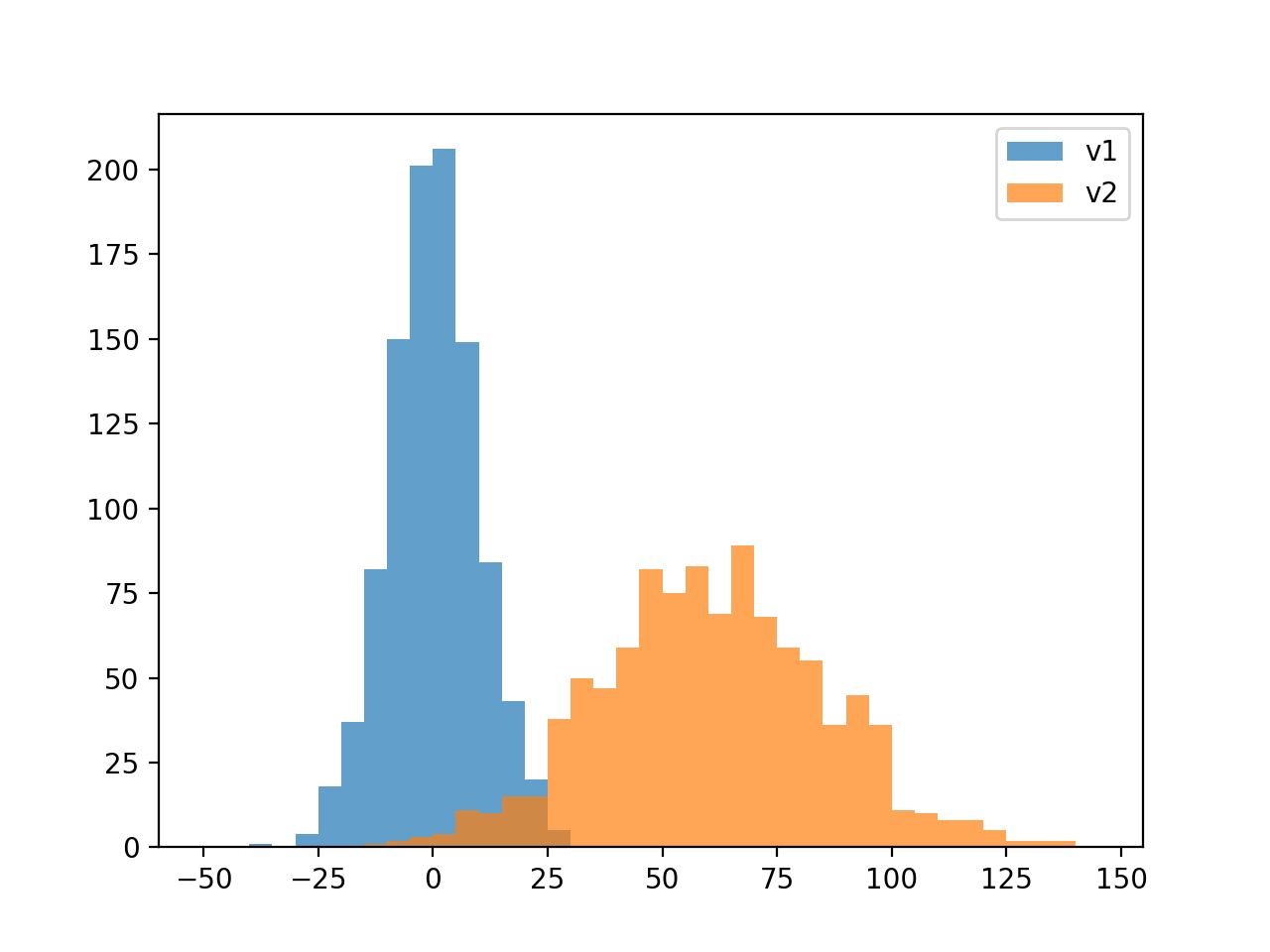In the following figure, the histograms are shown differently as a stacked bar plot. A kernel density estimate plot is placed over the stacked histogram. The kernel density estimation plot estimates the probability density function of the combination of the two series.

plt.figure()
plt.hist([v1, v2],
histtype='barstacked',
density=True);
v3 = np.concatenate((v1,v2))
sns.kdeplot(v3);

<IPython.core.display.Javascript object>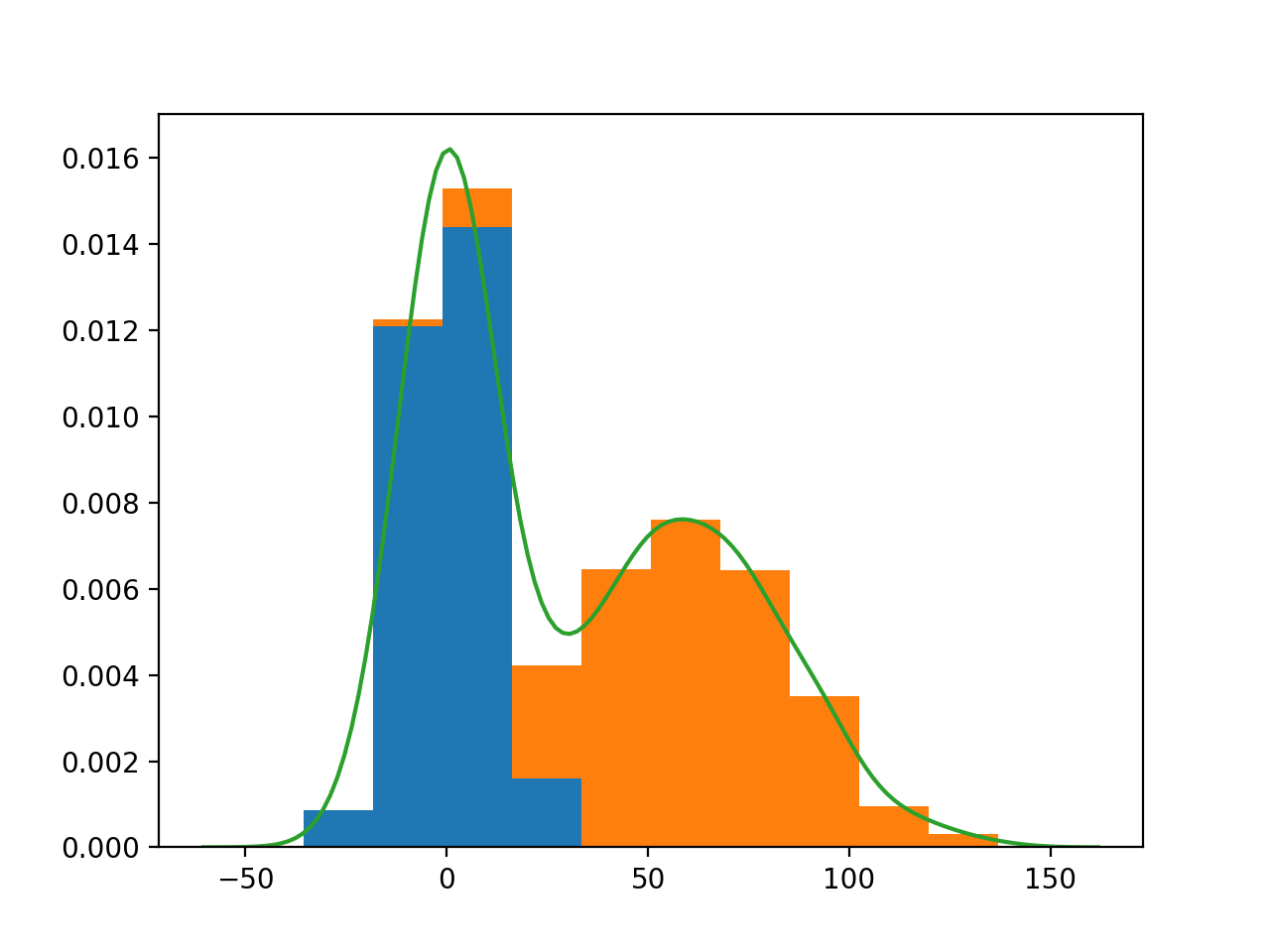Seaborn provides a function to quickly create this kind of plot called distplot.

plt.figure()
sns.distplot(v3, hist_kws={'color': 'Teal'}, kde_kws={'color': 'Navy'});

<IPython.core.display.Javascript object>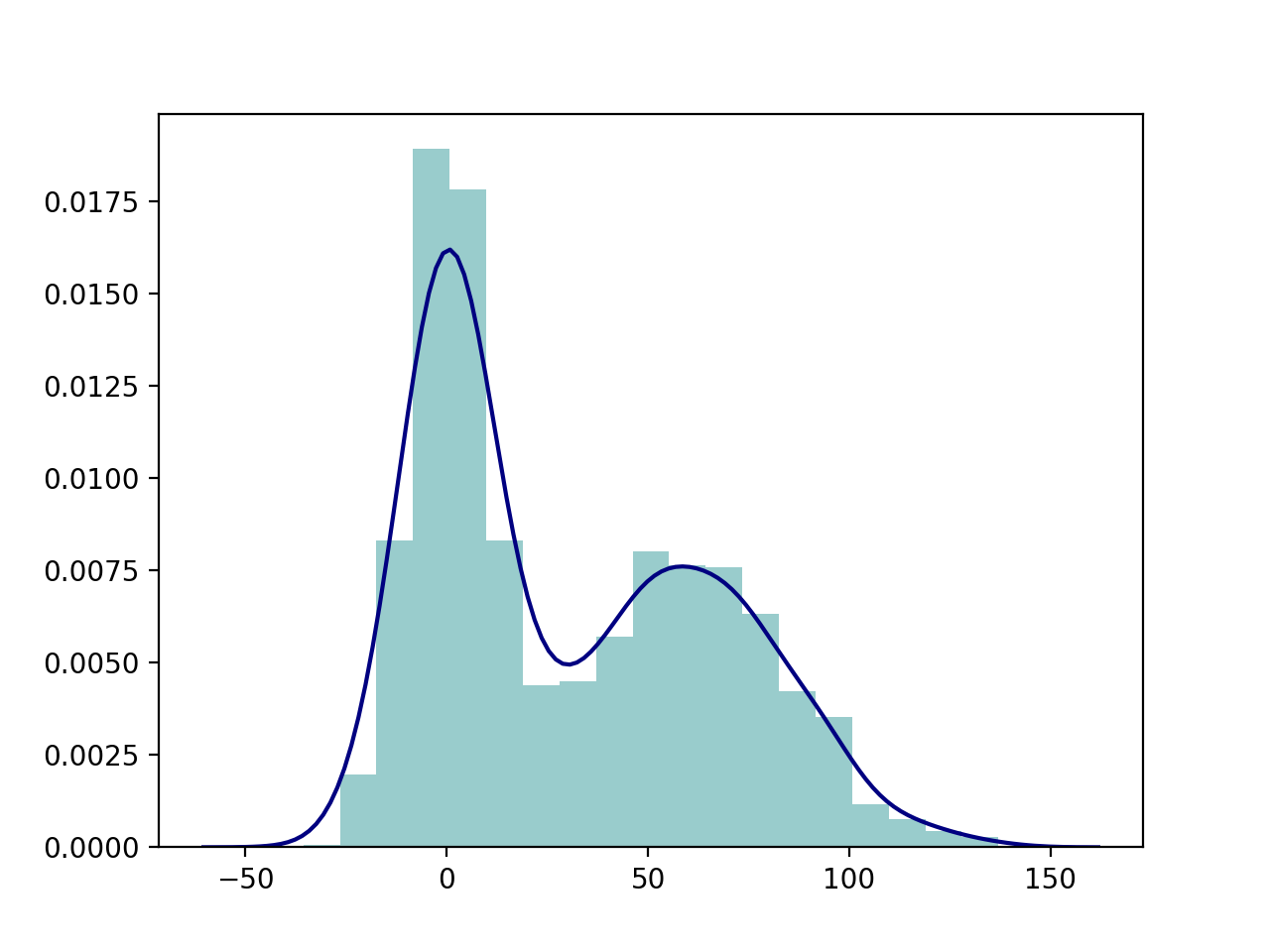The following is one of the complex plots sns contains built-in functions for, called a joint plot. It allows us to visualize the distribution of the two variables individually as histograms and jointly as a scatterplot.

sns.jointplot(v1, v2, alpha=0.4);

<IPython.core.display.Javascript object>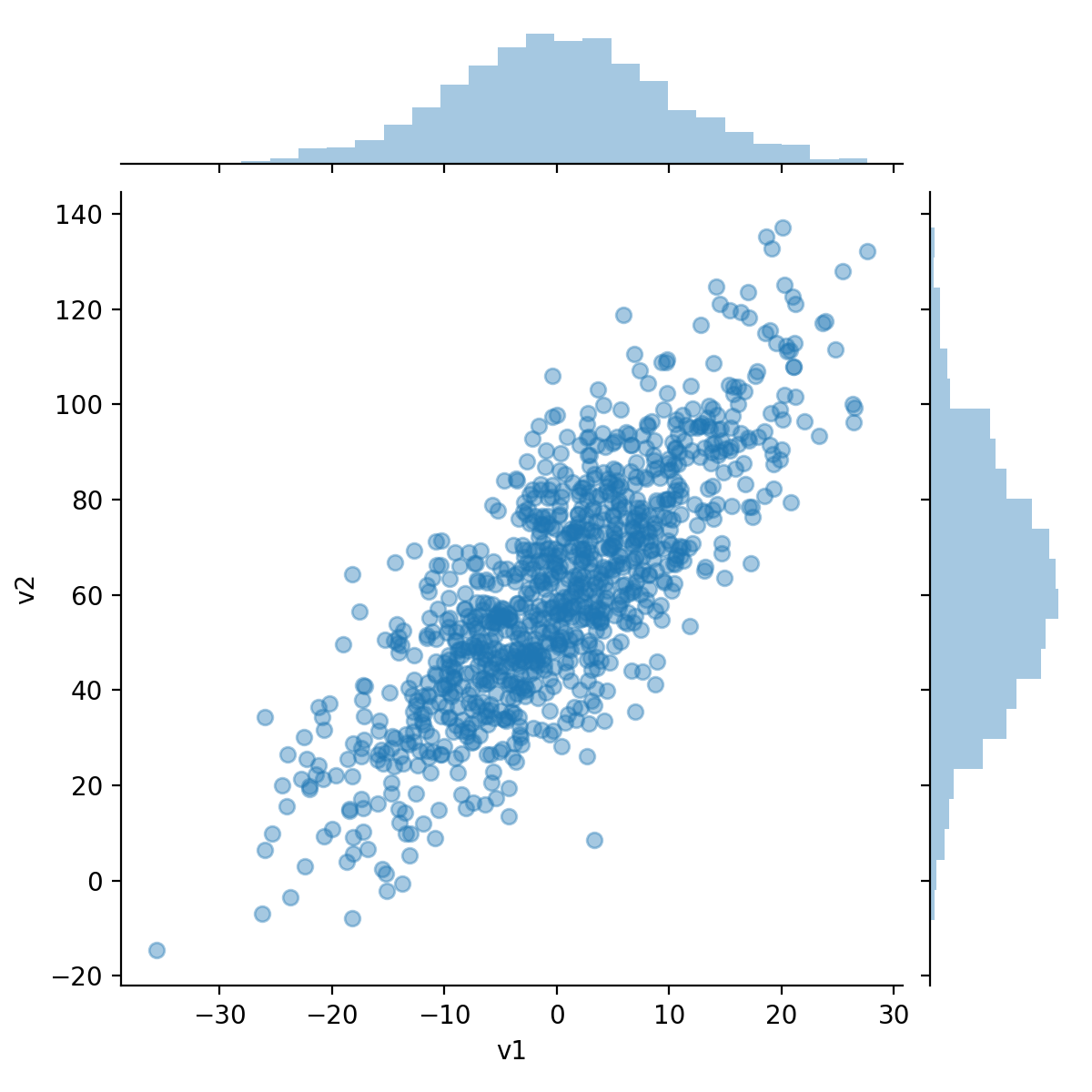Since Seaborn uses matplotlib we can change the plots using matplotlib’s tools. Some of Seaborn’s tools return a matplotlib axis object, while others return a Seaborn grid object which is a figure with several panels. jointplot falls into that category.

grid = sns.jointplot(v1, v2, alpha=0.4);
grid.ax_joint.set_aspect('equal')

<IPython.core.display.Javascript object>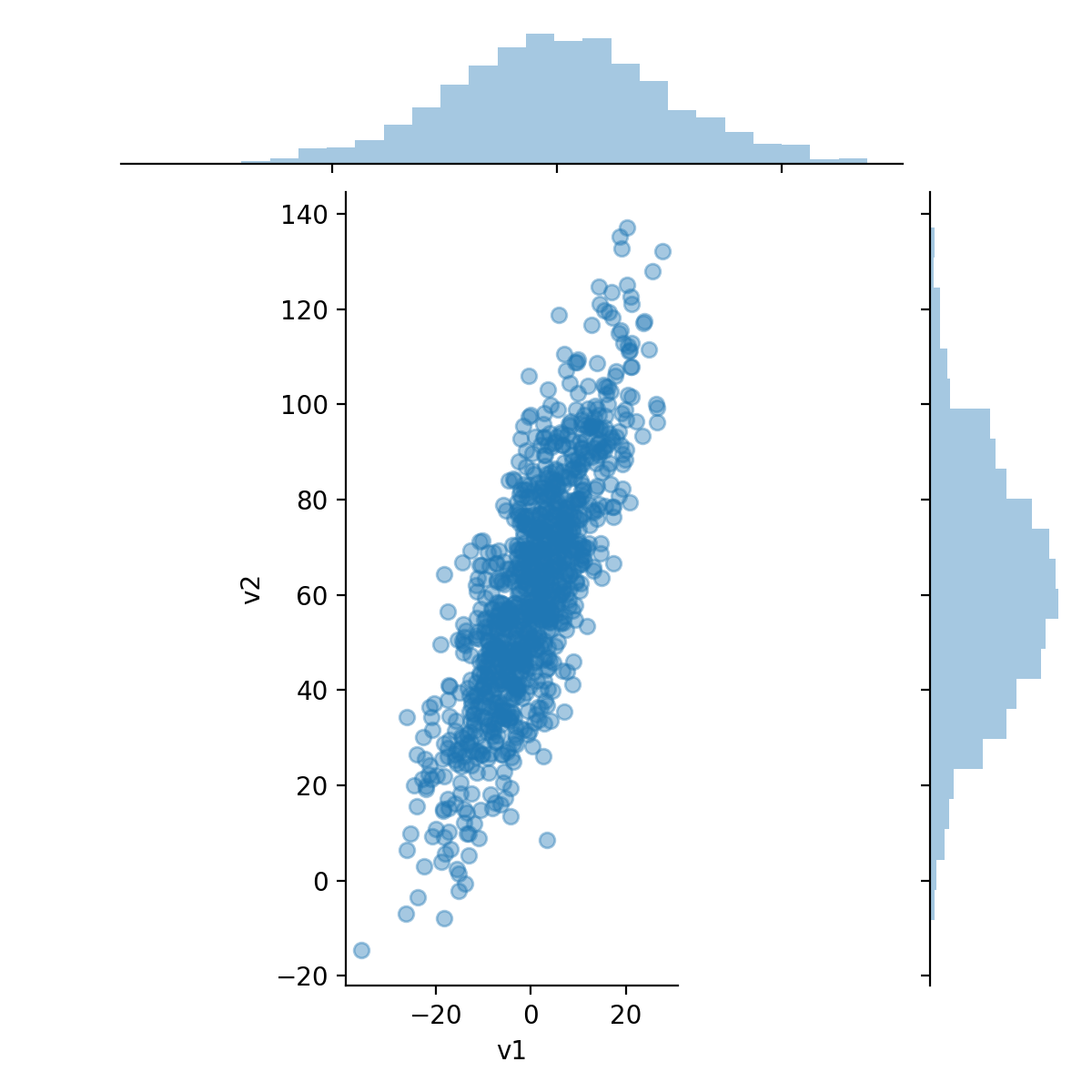Hexbin plots are the bivariate counterpart to histograms. They show the number of observations that fall into hexagonal bins. This type of plots works well with large datasets.

sns.jointplot(v1, v2, kind='hex');

<IPython.core.display.Javascript object>